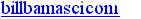TOP  | GOOD STUFF  | NEW STUFF  | SEARCH# Power Factor

```>Rob Sedgwick wrote at 11:38 AM 3/4/2013:
>
> Hi,
> I feel I have a valid and major concern regarding some universal energy
> measurement standards that are used in certain consumer devices,
> residential service meters and potentially (as my inquiry is yet
>
> Todays existing, typical energy consumption measurements use a watt-hour
> (Wh) or kilo-watt-hour (kWh) unit. Although the Wh or kWh unit is accurate
> for 100% linear (resistive) loads or the resistive portion of all loads,
> accurate and true measurements will need to calculate the total flow of
> electrons (amperage) through both the resistive and reactive portions of
>
> The reactive portion of inductive loads (most electronics, microwaves,
> fluorescent lights, refrigerators/freezers, pumps, etc) will increase the
> current flow to the load based on the power factor (electrical efficiency)
> increased current flow by improving the power factor.
> There is no 'free-lunch' when using the 'watt' consumption of a load and
> not accounting for any extra energy consumed from its inefficiency
```

Hi Rob!

Here's the central issue: Power Factor is not electrical efficiency.

The two are distantly related, but PF and efficiency are entirely different concepts. Because of Power Factor effects, any volt-amp meter measurements will often be wrong. Volt-amps gives an incorrect OVERestimation of the rate of actual energy consumption.

What's Power Factor? It's based on the idea that an (ideal) inductor consumes zero energy... yet whenever we plug inductors into AC outlets, the inductors draw significant current. (Same applies to capacitors.) If we multiply their voltage and current measurements, we'll see major watts of energy flow. But this wattage is wrong, and for ideal or non-lossy coils and capacitors, the actual energy consumption is zero.

The problem is that inductors do draw energy from the utility grid ...but then they turn around and give back every bit! During each cycle of AC, the electrical energy will be "sloshing" back and forth between the distant generator and the inductor. Resistors don't act like this. A resistor's current alternates, but the voltage alternates at the same time, so the energy flows in just one direction: from generator to load. With capacitors and coils, this doesn't happen.

Let's take a straightforward view which avoids imaginary numbers. We can investigate zero Power Factor by driving an inductor with a battery, then instantly swap the battery leads back and forth to create AC square waves. What happens?

[Diagram, four simple schematics 1a, 1b, 1c, 1d]

When first connected in [1a] above, the current rises smoothly, and the battery is dumping energy into the inductor as expected. In [1b] we flip the battery instantly. Since inductors act like "electricity flywheels" they have a large "inertia effect" on the flowing charges within them. The voltage polarity reverses, yet the direction of current does not. In [1b], the battery terminals have swapped, and the energy stored in the inductor flows back into the battery, charging it up.

If we leave the inductor connected, the current decreases, eventually goes through zero, and becomes negative, as in [1c]. The reversed battery voltage is driving a negative current, and negative times negative gives positive watts: this means that energy is again flowing from the battery into the inductor. Next, flip the battery again in [1d]. Again the current stays momentarily the same. But reversing the voltage has reversed the energy flow, and the inductor's stored energy is again being dumped back to the battery. Finally, wait until the current goes to zero, and we've created one complete "AC cycle."

See the problem? A large current exists in the power line, but our inductor uses up zero energy. On each half-cycle of AC, the inductor first draws some electrical energy from the power supply, but then it dumps it all back again. Volt-amperes remains large, yet energy consumption is zero, so PF is zero. We can't just look at volt-amperes, since it wrongly predicts that inductors consume immense energy. In fact, inductors consume very low energy (ideally it's zero.)

```>
> For the purpose of illustration, let's look at non-linear loads that
> basically have static consumptions (fluorescent lights, televisions).
> Let's neglect cyclic, variable or high starting loads such as
> refrigerators or pumps for now.
> I've used my 120VAC circuit measurement device (a standard tool on the
> appliance. This device is simply taking the measured_wattage_ of the
> appliance and multiplying it by the cumulative time recorded in the
> device. Although this same device has the capability to measure current,
> voltage and power factor it isn't using these values for proper energy
> consumption calculations.
> information for the consumer.
```

Nope, the energy meter is doing it right.

When connected to an inductor, it ignores the volt-amperes and the reversing energy flow. We don't want to measure the "sloshing energy" created by an inductor or a capacitor. We only care about the total energy which moves, on average, from generator to appliance. (And, if the capacitor or inductor is getting hot, that heat is caused by genuine one-way wattage being drawn from the power grid.)

```

```

```

```
http://amasci.com/elect/pfactor.html
Created and maintained by Bill Beaty. Mail me at:.
View My Stats